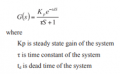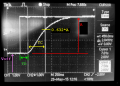# Control system

#### hero22222222222

Joined Dec 22, 2019
71

#### MrAl

Joined Jun 17, 2014
7,455
Hello there,

The parameters come from the drawing as shown in this modified drawing.
They then use Table 1 to get the values in Table 2.

If we ignore the offset, we get:
A*e^(-TD*s)/(TC*s+1)

If we include the offset we get:
A*e^(-TD*s)/(TC*s+1)+Voff/s

Where A, TD, TC and Voff are all are shown on the drawing.
Note this assumes (with high confidence) that the intersection marked "0.632*A" is the amplitude at that point where the horizontal yellow line and second vertical marker intersect the response curve.
It's very hard to tell from the drawing that TD=0.27 and TC=0.55 so those have to come from the text.

#### hero22222222222

Joined Dec 22, 2019
71
Hello there,

The parameters come from the drawing as shown in this modified drawing.
They then use Table 1 to get the values in Table 2.

If we ignore the offset, we get:
A*e^(-TD*s)/(TC*s+1)

If we include the offset we get:
A*e^(-TD*s)/(TC*s+1)+Voff/s

Where A, TD, TC and Voff are all are shown on the drawing.
Note this assumes (with high confidence) that the intersection marked "0.632*A" is the amplitude at that point where the horizontal yellow line and second vertical marker intersect the response curve.
It's very hard to tell from the drawing that TD=0.27 and TC=0.55 so those have to come from the text.

given thatand we aassume TD=0.27 and TC=0.55 and the amplitude of the responce is roughly 4.4V and the unit step has as amplitude of 4.4Vthen would the transfer function of this system (at its specific setting in time) be

[(1)(e^(-0.27s))]/(0.55s+1)

In addtion, if i wanted to simulate the exact practical step responce of this system. Would i multiply the transfer function with 4.4/s (unit step has amplitude of 4.4V in picture above) rather than 1/s then convert it to the time domain?

#### MrAl

Joined Jun 17, 2014
7,455
Hi,

Where are you getting this 4.4 from?
The amplitude can not be 4.4 so where do you see that?

Here is an example of simulating using LT Spice, using a dependent voltage source defined as a Laplace source.
LT Spice does not seem to accept the form e^at so we have to use exp(at) instead as you can see in the drawing.
The delay used for this example is 0.5 seconds. The amplitude is 1.
The delay causes that flat part of the response at the beginning. That flat part is exactly at zero. The "0.1" is actually "-0.1" but might be hard to read on some monitors.
Note the u(t) part of it is implied when we use the constant voltage source on the left to drive the Laplace source on the right.

Last edited:

#### hero22222222222

Joined Dec 22, 2019
71
Hi,

Where are you getting this 4.4 from?
The amplitude can not be 4.4 so where do you see that?

Here is an example of simulating using LT Spice, using a dependent voltage source defined as a Laplace source.
LT Spice does not seem to accept the form e^at so we have to use exp(at) instead as you can see in the drawing.
The delay used for this example is 0.5 seconds. The amplitude is 1.
The delay causes that flat part of the response at the beginning. That flat part is exactly at zero. The "0.1" is actually "-0.1" but might be hard to read on some monitors.
Note the u(t) part of it is implied when we use the constant voltage source on the left to drive the Laplace source on the right.
On the graph each y division is 1V so I got 4.4V

#### MrAl

Joined Jun 17, 2014
7,455
On the graph each y division is 1V so I got 4.4V
Hello again,

Ok, then shouldnt you include that in your transfer function? You have "1" there now.
I think once you add your gain value you then have what you need and that is the transfer function of whatever made that wave.

#### hero22222222222

Joined Dec 22, 2019
71
Hello again,

Ok, then shouldnt you include that in your transfer function? You have "1" there now.
I think once you add your gain value you then have what you need and that is the transfer function of whatever made that wave.
So the transfer function wouldn't change. All I would need to do is multiply the transfer function

[(1)(e^(-0.27s))]/(0.55s+1) by 4.4/s then convert to time domain to get the step response equal to the experimental result

#### MrAl

Joined Jun 17, 2014
7,455
So the transfer function wouldn't change. All I would need to do is multiply the transfer function

[(1)(e^(-0.27s))]/(0.55s+1) by 4.4/s then convert to time domain to get the step response equal to the experimental result
Hi,

Yes, unless there is an offset and then you have to add that to the response also.